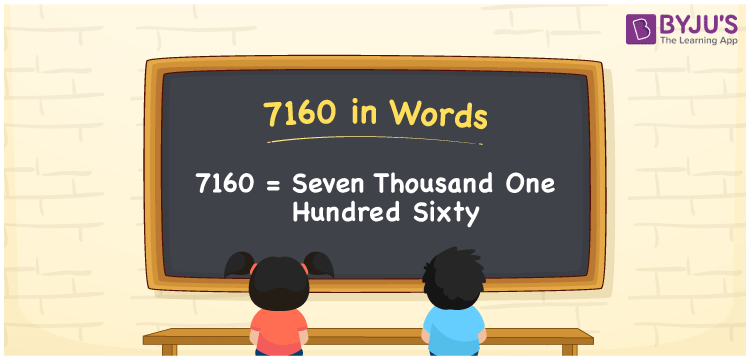# 7160 in Words

The number 7160 in words is written as Seven Thousand One Hundred Sixty. For instance, if the cost of a study table is Rs. 7160, then we can express it as, “The cost of a study table is Rupees Seven Thousand One Hundred Sixty”. 7160 is a cardinal number as it shows the specific quantity or value. The number 7160 can be converted into words easily using a place value chart. Learn the procedure to write the numerical name for the number 7160 using a place value system in this article.

 7160 in Words Seven Thousand One Hundred Sixty Seven Thousand One Hundred Sixty in numerical form 7160

## 7160 in English Words

We generally write the numbers in words with the help of the English alphabet. Therefore, we can read and write the number 7160 in English as Seven Thousand One Hundred Sixty.## How to Write 7160 in Words?

Conversion of numbers to words is easy using a place value system. The place value chart for the number 7160 is as follows.

 Thousands Hundreds Tens Ones 7 1 6 0

Therefore, we can write the expanded form as:

7 x Thousand + 1 x Hundred + 6 x Ten + 0 x One

= 7 x 1000 + 1 x 100 + 6 x 10 + 0 x 1

= 7000 + 100 + 60 + 0

= 7000 + 100 + 60

= 7160

= Seven Thousand One Hundred Sixty

Hence, 7160 in words is written as Seven Thousand One Hundred Sixty

Interesting way of writing 7160 in words

7 = Seven

71 = Seventy-One

716 = Seven Hundred and Sixteen

7160 = Seven Thousand One Hundred Sixty

Thus, the word form of the number 7160 is Seven Thousand One Hundred Sixty

7160 is a natural number that precedes 7161 and succeeds 7159

• 7160 in words – Seven Thousand One Hundred Sixty
• Is 7160 an odd number? – No
• Is 7160 an even number? – Yes
• Is 7160 a perfect square number? – No
• Is 7160 a perfect cube number? – No
• Is 7160 a prime number? – No
• Is 7160 a composite number? – Yes

## Frequently Asked Questions on 7160 in Words

Q1

### How to write 7160 in words?

The number 7160 in words is Seven Thousand One Hundred Sixty.
Q2

### Simplify 6000 + 1160, and express in words.

Simplifying 6000 + 1160, we get 7160. The number 7160 in words is Seven Thousand One Hundred Sixty.
Q3

### How to write Seven Thousand One Hundred Sixty in numbers?

Seven Thousand One Hundred Sixty in numbers is 7160.